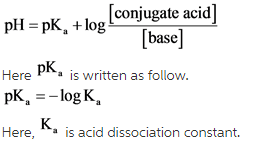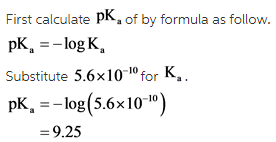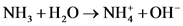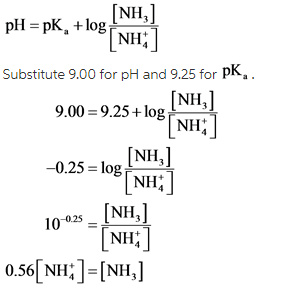# What mass of ammonium chloride, NH4Cl, (Ka = 5.6 x 10^-10) must be added to exactly 500mL of 0.10M

What mass of ammonium chloride, NH4Cl, (Ka = 5.6 x 10^-10) must be added to exactly 500mL of 0.10M NH3 solution to give a solution with a pH of 9.00?

Concepts and reason
The concept used to solve this problem is based on the chemical equilibrium.
The pH of solution is used to determine acidity and basicity of a solution.

Fundamentals
The pH of solution can be determine by Henderson-Hasselbalch equation as follow.